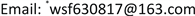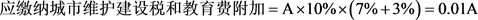﻿ 房地产企业增值税纳税筹划思路探究 Research on the Thoughts of Value Added Tax Planning of Real Estate Enterprises

Frontiers of International Accounting
Vol. 08  No. 01 ( 2019 ), Article ID: 28823 , 7 pages
10.12677/FIA.2019.81003

Research on the Thoughts of Value Added Tax Planning of Real Estate Enterprises

Lei Shang, Shufeng Wang*

College of Accounting, Heilongjiang Bayi Agriculture University, Daqing HeilongjiangReceived: Jan. 21st, 2019; accepted: Feb. 5th, 2019; published: Feb. 12th, 2019ABSTRACT

The real estate industry has long been a leading economic growth point among various industries in China. Under the national macro-control and the regulation of the market itself, the overall taxation level has gradually tended to be stable. Due to the nature of real estate development enterprises, they need a large amount of capital investment in the process of operation. It is particularly important to be able to plan tax payment business on the basis of business planning. Therefore, by means of comparative analysis, judgment and reasoning, case analysis and other methods, tax planning is carried out around the value added tax issues involved in the relevant business of real estate business operations, in order to put forward some new ideas for value added tax planning for similar enterprises.

Keywords:Real Estate Enterprise, Value Added Tax Planning, Tax Balance PointCopyright © 2019 by authors and Hans Publishers Inc.

This work is licensed under the Creative Commons Attribution International License (CC BY).

http://creativecommons.org/licenses/by/4.0/1. 引言

2. 房地产企业增值税筹划的原则与目标

2.1. 房地产企业增值税筹划的现状

2.2. 房地产企业增值税筹划目标

3. 房地产企业增值税筹划具体思路

3.1. 进项税额的筹划

3.1.1. 强化采购发票审核管理

3.1.2. 灵活选择采购合作方

1) 小规模纳税人作为供应商

$应缴增值税=\text{A}×\text{1}0%=0.\text{1A}$$应缴纳所得税=\left(\text{A}-{\text{W}}_{\text{1}}-0.0\text{1A}\right)×\text{25}%=0.\text{2475A}-0.{\text{25W}}_{\text{1}}$

$税后净利润=\left(\text{A}-{\text{W}}_{\text{1}}-0.0\text{1A}\right)×\left(\text{1}-\text{25}%\right)=0.\text{7425A}-0.{\text{75W}}_{\text{1}}$

${\text{T}}_{\text{1}}=0.\text{1A}-+0.0\text{1A}+0.\text{2475A}-0.{\text{25W}}_{\text{1}}=0.\text{3575A}-0.{\text{25W}}_{\text{1}}$

2) 一般纳税人作为供应商

$应缴增值税=\text{A}×\text{1}0%-{\text{W}}_{\text{2}}×\text{16}%=0.\text{1A}-0.{\text{16W}}_{\text{2}}$

$应纳城建税和教育费附加=\left(0.\text{1A}-0.{\text{16W}}_{\text{2}}\right)×\left(\text{7}%+\text{3}%\right)=0.0\text{1A}-0.0{\text{16W}}_{\text{2}}$

$应缴纳所得税=\left[\text{A}-{\text{W}}_{\text{2}}-\left(0.0\text{1A}-0.0{\text{16W}}_{\text{2}}\right)\right]×\text{25}%=0.\text{2475A}-0.{\text{246W}}_{\text{2}}$

$税后净利润=\left[\text{A}-{\text{W}}_{\text{2}}-\left(0.0\text{1A}-0.0{\text{16W}}_{\text{2}}\right)\right]×\left(\text{1}-\text{25}%\right)=0.\text{7425A}-0.{\text{738W}}_{\text{2}}$

$\begin{array}{l}{\text{T}}_{\text{2}}=0.\text{1A}-0.{\text{16W}}_{\text{2}}+0.0\text{1A}-0.0{\text{16W}}_{\text{2}}+0.\text{2475A}-0.{\text{246W}}_{\text{2}}\\ \text{\hspace{0.17em}}\text{\hspace{0.17em}}\text{\hspace{0.17em}}\text{\hspace{0.17em}}=0.\text{3575A}-0.{\text{422W}}_{\text{2}}\end{array}$

3) 税负影响下物资采购价格平衡点

3.1.3. 劳务用工费抵扣的筹划

3.2. 进项税额转出的筹划

1) 直接计入仓库施工项目的工程成本

2) 先抵扣后转出

3.3. 销项税额的筹划

3.3.1. 合理选择销售模式

3.3.2. 适当分解租金收入

$应缴增值税=1500×10%-35×6%-20×10%-65×16%=\text{135}.\text{5}万元$

$应缴纳城市维护建设税和教育费附加=135.5×\left(3%+7%\right)=13.55万元$

$应缴房产税=\text{15}00×\text{12}%=\text{18}0万元$

$应缴所得税=\left(\text{15}00-\text{2}0-\text{65}-\text{13}.\text{55}-\text{18}0\right)×\text{25}%=\text{3}0\text{5}.\text{36}万元$

$税后净利润=\left(\text{15}00-\text{2}0-\text{65}-\text{13}.\text{55}-\text{18}0\right)×\left(\text{1}-\text{25}%\right)=\text{916}.0\text{9}万元$

$应缴增值税=1380×10%+35×6%=140.1万元$

$应缴纳城市维护建设税和教育费附加=\text{14}0.\text{1}×\left(\text{3}%+\text{7}%\right)=\text{14}.0\text{1}万元$

$应缴房产税=\text{138}0×\text{12}%=\text{165}.\text{6}万元$

$应缴所得税=\left(\text{138}0+\text{35}-\text{14}.0\text{1}-\text{165}.\text{6}\right)×\text{25}%=\text{3}0\text{8}.\text{85}万元$

$税后净利润=\left(\text{138}0+\text{35}-\text{14}.0\text{1}-\text{165}.\text{6}\right)×\left(\text{1}-\text{25}%\right)=\text{926}.\text{54}万元$

3.3.3. 买房送车销售业务的筹划

4. 结论

Research on the Thoughts of Value Added Tax Planning of Real Estate Enterprises[J]. 国际会计前沿, 2019, 08(01): 13-19. https://doi.org/10.12677/FIA.2019.81003

1. 1. 王树锋, 路博文. 视同销售行为增值税纳税筹划风险及危害[J]. 财会月刊, 2015(10): 115-118.

2. 2. 张伟泽. 房地产企业税收筹划问题研究[D]: [硕士学位论文]. 大庆: 黑龙江八一农垦大学, 2016.

3. 3. 王树锋, 冯凌. 营改增环境下房地产企业增值税筹划方法研究[J]. 国际会计前沿, 2017, 6(2): 5-9.

4. 4. 李强, 倪云珍, 王树锋. 建筑业增值税纳税筹划要点研究[J]. 商业会计, 2017(9): 121-123.

5. 5. 张驰. 山东鸿顺建筑公司增值税纳税筹划研究[D]: [硕士学位论文]. 大庆: 黑龙江八一农垦大学, 2016.

6. 6. 张楷笛. 后“营改增”时代LH房地产企业增值税纳税筹划探讨[D]: [硕士学位论文]. 青岛: 青岛理工大学, 2018.

NOTES

*通讯作者。